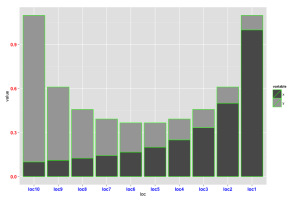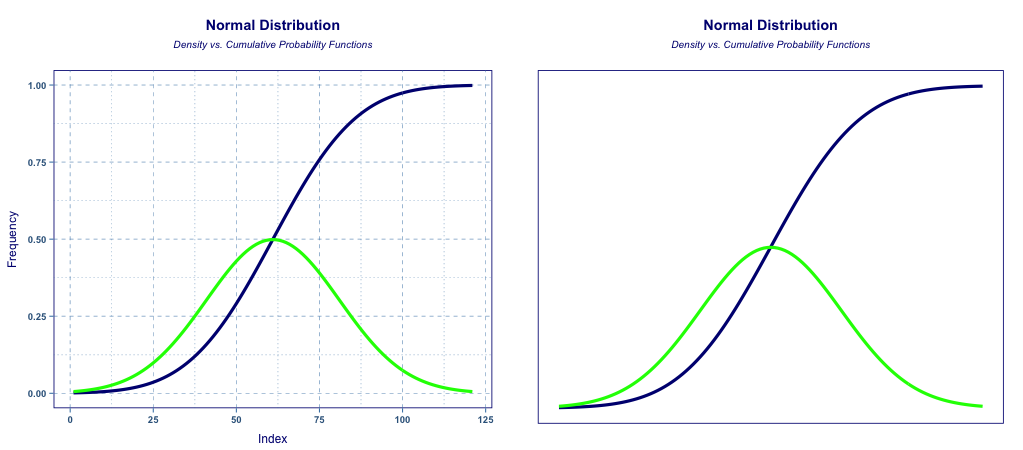# Axes (ggplot)

#### Data

A simple sequence of numbers is provided to populate the base graph.

#### Axes Text Formats

The following examples modify axes text formats with colors and bold font so they are easier to see.  The second chart benefits from axis text being rotated 90 degrees.

#### Axes Range

Controlling the range of values on the y axis is straightforward.  For some graph types, care is required as data outside the axis range can be lost.  This problem can be avoided by using the coord_cartesian , as shown below, which simply zooms the view within the existing graph device (as opposed to reconfiguring the device itself).

#### Axes Tick Intervals

Controlling the tick mark intervals is a function of the range and range breaks, as shown below.  Ticks can also be suppressed or removed.  One example shown below removes tcp intervals and gridlines, while the other leaves gridlines intact.

#### Axes Tick Number Formats

Tick number formats can be set to percent, currency, comma-delimited, or scientific formats using formatter functions from the scales package.

Axis scales over 1,000, can be formatted with labels = comma.  Dates can be formatted using labels = date_format(format = %Y%m%d), where the format input code uses standard POSIX specifications (as defined here).

#### Changing Axes Item Order#### Axes Transforms: Standard vs. Custom Functions

Linear scaling of the axes is the default behavior of the R graphic devices.  However function conversions are also possible, such as log10, power functions, square root, logic, etc.  There are four ways to convert or rescale an axes:

• transform the data being plotted;
• transform the axis using a standard scale transform such as scale_y_log10(),
• transform the coordinate system of the graphic device with coord_trans(),
• create a custom transformation function with trans_new().

The first method, manual transforms of the data, is straightforward.  Axis scale transforms also convert the data using internal functions.   The primary benefit is that data is changed before chart properties are affected, preserving the ability to call axes control options (such as breaks and break labels).  The disadvantage is that the number of predefined scale functions is limited.  Coordinate transforms are “different to scale transformations” according to ggplot documentation since conversion of data occurs after chart options are set, reducing flexibility.  Finally, custom functions can be defined using trans_new().  In this case a function is defined by the user using the naming convention myfun_trans.  Arguments then require the call name (myfun), the transform function and the inverse function.  Examples appear below.

#### Removing Axis Gridlines, Ticks, Titles and Labels

The theme function can be used to quickly eliminate axis elements.  The example below shows before and after: